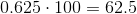## Example Questions

### Example Question #1 : How To Find Percentage Equivalent To A Decimal

Choose the answer below which best expresses the following decimal as a percent: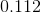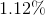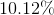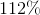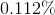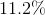Explanation:

To convert a percent into a decimal, simply know that the tenths place of the decimal will correspond to the tens digit of the percent, and the thousandths place of the decimal will correspond to the ones digit of the percent. Any ensuing digits will be behind a decimal in the final percent.

Also converting a decimal to a percent can be accomplished by multiplying the percent by 100.

Therefore, the best answer here is: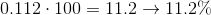### Example Question #2 : How To Find Percentage Equivalent To A Decimal

Choose the answer which best expresses the following decimal as a percentage, rounded to the nearest tenth of a percent if necessary: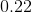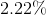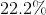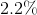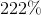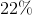Explanation:

To express a decimal as a percent, multiply the decimal by one hundred: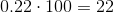### Example Question #1 : Decimals And Percentage

Choose the answer which best expresses the following decimal as a percentage, rounded to the nearest tenth of a percent if necessary: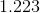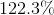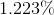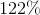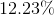Explanation:

To express a decimal as a percent, multiply the decimal by one hundred: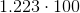### Example Question #4 : How To Find Percentage Equivalent To A Decimal

Choose the answer which best expresses the following decimal as a percentage, rounded to the nearest tenth of a percent if necessary: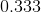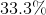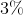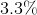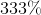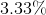Explanation:

To express a decimal as a percent, multiply the decimal by one hundred: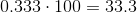### Example Question #5 : How To Find Percentage Equivalent To A Decimal

Choose the answer which best expresses the following decimal as a percentage, rounded to the nearest tenth of a percent if necessary: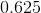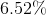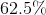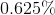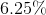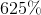Explanation:

To find the equivalent percentage we need to multiply the decimal by 100.